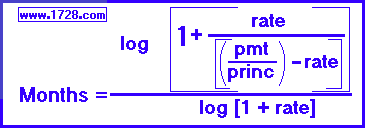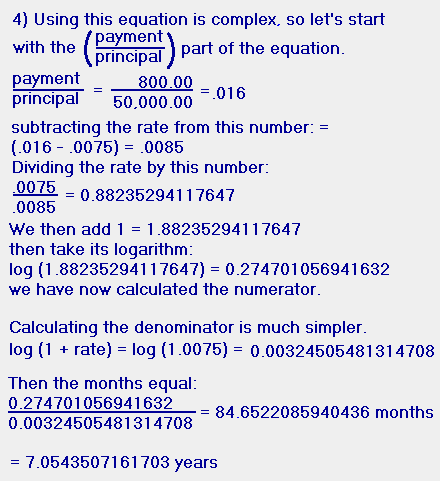Loan Payment Formula - YearsWhere rate = Annual Percentage Rate divided by 1200
For example, an 8 % annual rate becomes .0066666666666666...

 Here, the loan formula has been solved for months. As you can see from the above formula, solving for months is a bit trickier than solving for monthly payment or principal. We need to take a loan for \$50,000 and we can afford to pay \$800.00 per month. The local bank charges 9 per cent interest. How much time will it take to repay this loan? 1) The rate would be 9 ÷ 1,200 = .0075 2) The principal is \$50,000.00 3) The monthly payment is \$800.00RETURN   TO   HOME   PAGE